### Double Revolving Field Theroy

According to this theory, any alternating quantity can be resolved into two rotating components which rotate in opposite directions and each having magnitude as half of the maximum magnitude of the alternating quantity.
In case of single phase induction motors, the stator winding produces an alternating magnetic field having maximum magnitude of Φ1m.
According to double revolving field theory, consider the two components of the stator flux, each having magnitude half of maximum magnitude of stator flux i.e. (Φ1m/2). Both these components are rotating in opposite directions at the synchronous speed Ns which is dependent on frequency and stator poles.
Let Φ is forward component rotating in anticlockwise direction while Φ is the backward component rotating in clockwise direction. The resultant of these two components at any instant gives the instantaneous value of the stator flux at the instant. So resultant of these two is the original stator flux.Fig. 1  Stator flux and its two components
The Fig. 1 shows the stator flux and its two components Φand Φb. At start both the components are shown opposite to each other in the Fig.1(a). Thus the resultant ΦR = 0. This is nothing but the instantaneous value of the stator flux at start. After 90o , as shown in the Fig. 1(b), the two components are rotated in such a way that both are pointing in the same direction. Hence the resultant ΦR is the algebraic sum of the magnitudes of the two components. So ΦR = (Φ1m/2) + (Φ1m/2) =Φ1m. This is nothing but the instantaneous value of the stator flux at θ = 90o as shown in the Fig 1(c). Thus continuous rotation of the two components gives the original alternating stator flux.
Both the components are rotating and hence get cut by the motor conductors. Due to cutting of flux, e.m.f. gets induced in rotor which circulates rotor current. The rotor current produces rotor flux. This flux interacts with forward component Φto produce a torque in one particular direction say anticlockwise direction. While rotor flux interacts with backward component Φb to produce a torque in the clockwise direction. So if anticlockwise torque is positive then clockwise torque is negative.
At start these two torque are equal in magnitude but opposite in direction. Each torque tries to rotate the rotor in its own direction. Thus net torque experienced by the rotor is zero at start. And hence the single phase induction motors are not self starting.

Torque speed characteristics
The two oppositely directed torques and the resultant torque can be shown effectively with the help of  torque-speed characteristics. It is shown in the Fig.2.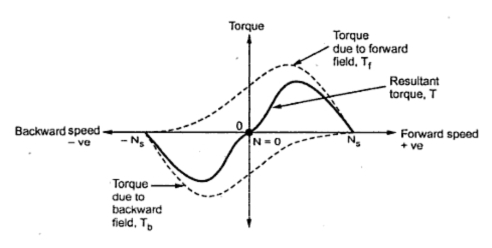Fig. 2  Torque-speed characteristic

It can be seen that at start N = 0 and at that point resultant torque is zero. So single phase motors are not self starting.
However if the rotor is given an initial rotation in any direction, the resultant average torque increase in the direction in which rotor initially rotated. And motor starts rotating in that direction. But in practice it is not possible to give initial torque to rotor externally hence some modifications are done in the construction of single phase induction motors to make them self starting.
Another theory which can also be used to explain why single phase induction motors is not self starting is cross-field theory.

1.2.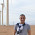excellent explanation

3.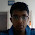thanks good explanation

4.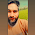Nice solution

5.Very good explanation

6.Good explanation

7.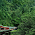8.Nice explain. thanks

1.Nice explanation